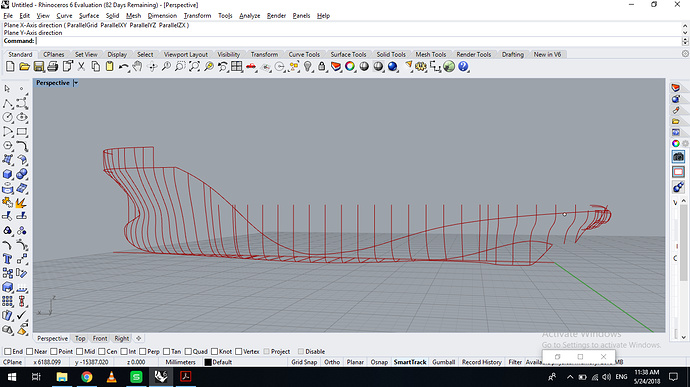# Equation of NURBS curve in Rhino

Hi!
I have station coordinates(offset table) of a ship. It is of around 75m length and I want to get all station curve equation so that I can scale my ship from 60-100m length and corresponding breadth and depth. How can I get the NURBS equation of all the stations so I can scale them properly.
Following is the screenshot of the station curves.Hi @vishalg2235,

As mentioned in the other post - you need to define NURBS and use curves as basis.
Afterwards - scaling can be done.

As rhino joins the points with a NURBS curve so is there a way I can extract that NURBS equation? So that I can input that equation for scaled coordinates as nature of station curve will be same.

@Dmitriy Maybe I’am not getting your point can you please elaborate. How can I define a NURBS curve? Rhino itself is defining it from points.

Hi,
I was referring to the surfaces based on NURBS.
You don’t need equations if you just need simple scaling.

Do you still need to calculate hydrostatics?

Yes. I want to calculate hydrostatic curves of all the scaled ships.
But was planning to do it in Maxsurf as after scaling points in Excel I will have all the coordinates.

So, then you need to model surfaces anyway.
Now you have curves and you need to use them as basis for surfaces.

No in maxsurf ship’s hull can be straightaway generated by meshing the points. So that’s why I think I only need the points of scaled ship.

Ok, are you going to use Maxsurf for Hydrostatics?
Have not used Maxsurf for some time now but if I remember - it cannot handle properly creases/knuckle curves.
As I see it from your shape - you have some knuckles in the aft.

I have no experience of Maxsurf but I found it on web videos that it can do so. But most importantly the stability calculations will be done very fast in it. Yes. There is a knuckles in aft and will try to remove it in maxsurf only.
Can you tell me the way how can I get those curve equations?

Hello @vishalg2235,
Again: you don’t need equation if you need to perform simple scaling of the hull.

So, now - are you talking about Stability calculations, which should involve calculation of the GZ lever arm curve with heel as an argument or simply hydrostatics?

Maxsurf actually uses NURBS surfaces similar to Rhino but Rhino is much more flexible when comes to preparing of complicated hulls with trimmed surfaces, knuckles, tunnels and etc.

If you can do everything in Excel and Maxsurf - why do you need to use Rhino then?

Kind regards,
Dmitriy